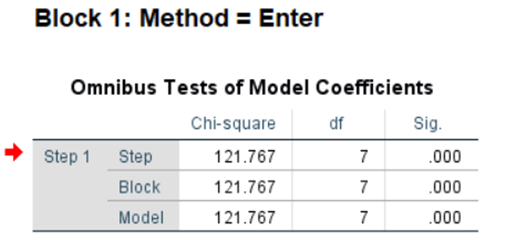# Binary Logistic Regression using SPSS

## What is it All About?

Logistic regression analysis is a method to determine the reason-result relationship of independent variable(s) with dependent variable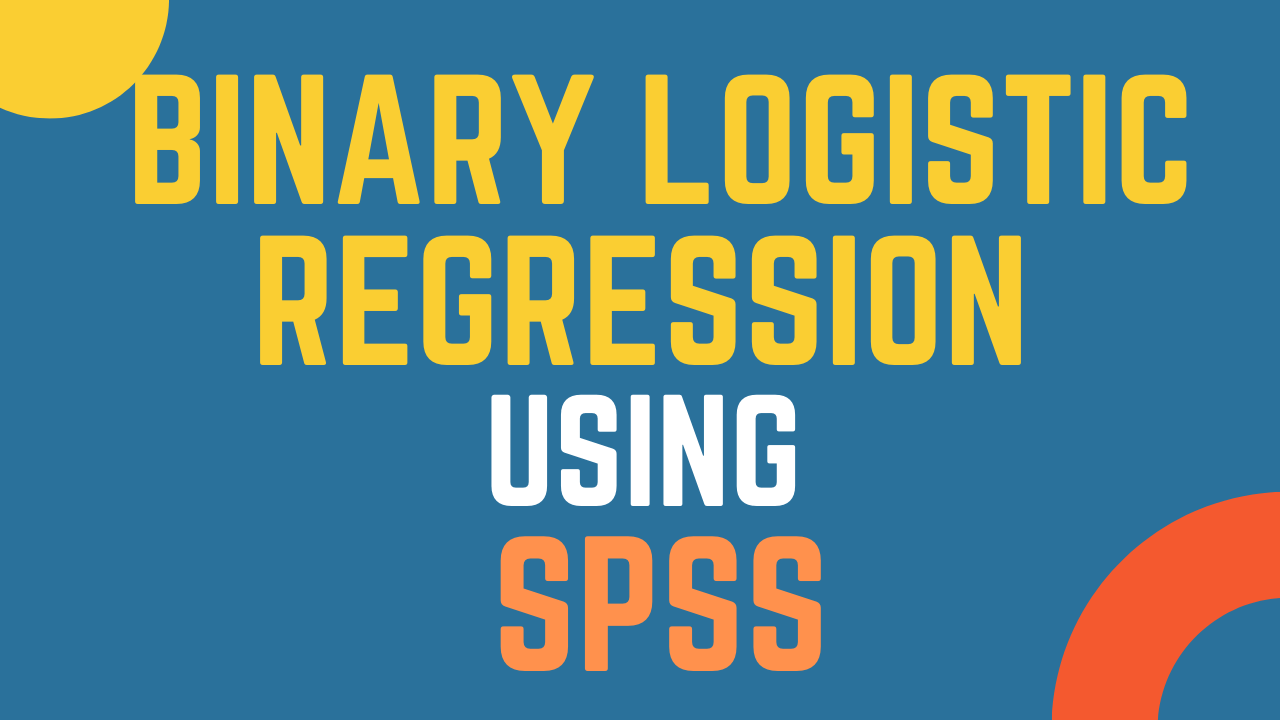### Learn to Analyze Data using Binary Logistic Regression using SPSS

The tutorial is a step by step guide on how to perform Binary Logistic Regression using SPSS.

## The Concept of Binary Logistic Regression

#### "What to do when we have a binary/Dichotomous outcome variable in Research"

Regression technique is used to assess the strength of a relationship between one dependent and independent variable(s). It helps in predicting value of a dependent variable from one or more independent variable. Regression analysis helps in predicting how much variance is being accounted in a single response (dependent variable) by a set of independent variables.

Linear regression analysis requires the outcome/criterion variable to be measured as a continuous variable. (To Learn More About Regression Analysis, Click Here). However, there may be situations when the researcher would like to predict an outcome that is dichotomous/binary.

In such situation, a scholar can use Binary Logistic Regression to assess the impact of one of more predictor variables on the outcomes. Logistic regression analysis is a method to determine the reason-result relationship of independent variable(s) with dependent variable

The logistic regression predicts group membership

• Since logistic regression calculates the probability of success over the probability of failure, the results of the analysis are in the form of an odds ratio.
• Logistic regression determines the impact of multiple independent variables presented simultaneously to predict membership of one or other of the two dependent variable categories.
• In logistic regression, the expected outcome is represented by 1 while the other is coded as 0.

## Examples of Binary Logistic Regression

#### A scholar may utilize binary logistic regression in following situations

• A store would like to assess factors that lead to return/no return of the customer.
• Assess if a particular candidate wins/loses an election based on the time spent in constituency, previously elected, no. of issues resolved.
• A HR researcher would like to ascertain how factors like experience, years of education, previous salary, university ranking affect the selection of a candidate in a job interview.
• A scholar would like to predict the choice of bank (Public or Private) based on independent variables that include Technology, Interest Rates, Value Added Services, Perceived Risks, Reputation, and others.

## Assumptions

• Logistic regression does not assume a linear relationship between the dependent and independent variables.
• The independent variables need not be interval, nor normally distributed, nor linearly related, nor of equal variance within each group
• Homoscedasticity is not required. The error terms (residuals) do not need to be normally distributed.
• The dependent variable in logistic regression is not measured on an interval or ratio scale. The dependent variable must be a dichotomous ( 2 categories) for the binary logistic regression.
• The categories (groups) as a dependent variable must be mutually exclusive and exhaustive; a case can only be in one group and every case must be a member of one of the groups.
• Larger samples are needed than for linear regression because maximum coefficients using a ML method are large sample estimates. A minimum of 50 cases per predictor is recommended (Field, 2013)
• Hosmer, Lemeshow, and Sturdivant (2013) suggest a minimum sample of 10 observations per independent variable in the model, but caution that 20 observations per variable should be sought if possible.
• Leblanc and Fitzgerald (2000) suggest a minimum of 30 observations per independent variable.

## Example Problem

For the purpose of this tutorial, i am considering the following example. The IVs are on interval scale while the DV is binary (Public or Private Bank)

• A scholar would like to predict the choice of bank (Public or Private) based on independent variables that include Technology, Interest Rates, Value Added Services, Perceived Risk, Reputation, Attractiveness, and Perceived Costs.
The scholar is interest in predicting the odds of selection of Public or Private bank on the perception of respondents in relation to the factors like Technology, Interest Rates, Value Added Services, Perceived Risk, Reputation, Attractiveness, and Perceived Costs.
For instance, the scholar hypothesized, that improved technology, offering better interest rates, improved value added services and other predictors will lead to choosing Private banks for account opening.

## How to Run Binary Logistic Regression

Step 1: In SPSS, Go to Analyze -> Regression -> Binary Logistic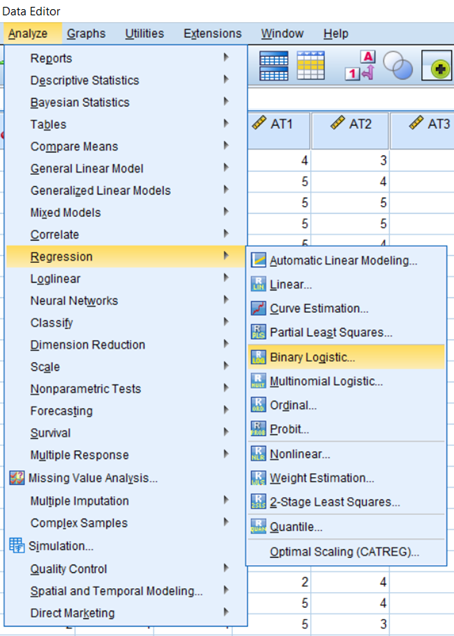Step 2: Next, The Logistic Regression Dialog Box will Appear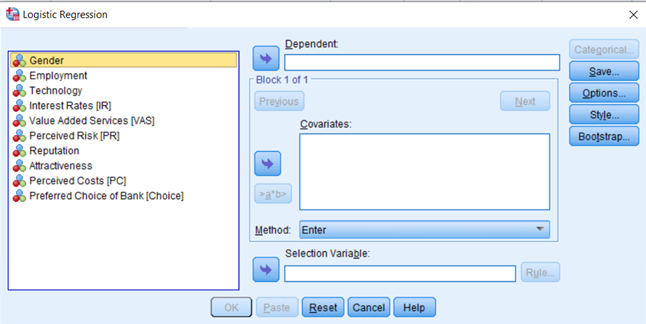Step 3: Add Preferred Choice of Bank [Choice] in the Dependent Box and Add IVs, Technology, Interest Rates, Value Added Services, Perceived Risk, Reputation, Attractiveness, and Perceived Costs in the Covariates list box.The Dialog box should now look like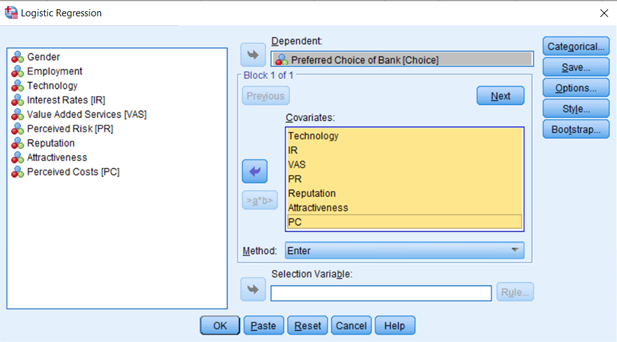Step 4: Next, Select Options, Check, Hosmer-Lemeshow goodness-of-fit and CI for exp(B)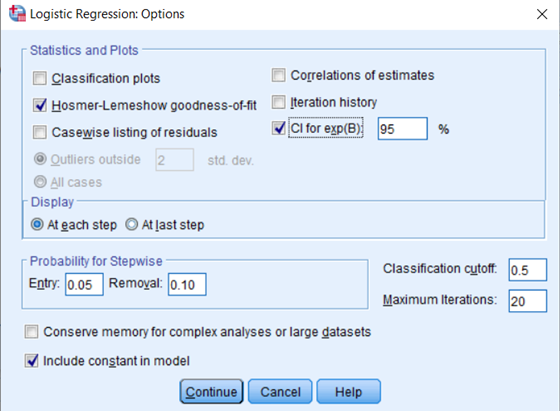Step 5: Press, Continue, and then Press OK.

## Interpreting Binary Logistic Regression

### Case Processing Summary and Encoding

The first section of the output shows Case Processing Summary highlighting the cases included in the analysis. In this example we have a total of 341 respondents.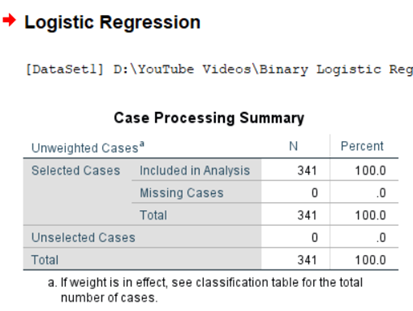The Dependent variable encoding table shows the coding for the criterion variable, in this case those who will encourage are classified as 1 while those who will not encourage to take up the Islamic Banking are classified as 0.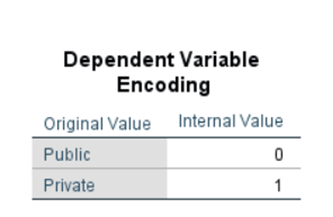### Block 0

The next section of the output, headed Block 0, is the results of the analysis without any of our independent variables used in the model. This will serve as a baseline later for comparing the model with our predictor variables included.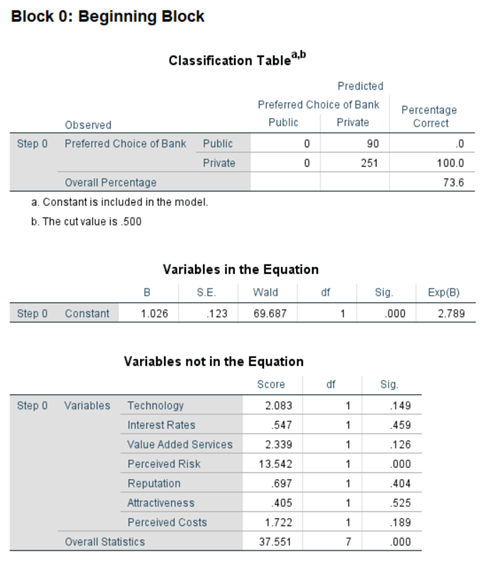### Block 1: Method = Enter

Omnibus Tests of Model Coefficients is used to test the model fit. If the Model is significant, this shows that there is a significant improvement in fit as compared to the null model, hence, the model is showing a good fit.